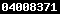QUANTITATIVE ELECTRONIC STRUCTURE - ACTIVITY RELATIONSHIPS ANALYSIS ANTIMUTAGENIC BENZALACETONE DERIVATIVES BY PRINCIPAL COMPONENT REGRESSION APPROACHhttps://doi.org/10.22146/ijc.21876

Yuliana Yuliana(1*), Harno Dwi Pranowo(2), Jumina Jumina(3), Iqmal Tahir(4)

(3) Chemistry Department, Faculty of Mathematics and Natural Sciences, Universitas Gadjah Mada, Yogyakarta
(*) Corresponding Author

Abstract

Quantitative Electronic Structure Activity Relationship (QSAR) analysis of a series of benzalacetones has been investigated based on semi empirical PM3 calculation data using Principal Components Regression (PCR). Investigation has been done based on antimutagen activity from benzalacetone compounds (presented by log 1/IC50) and was studied as linear correlation with latent variables (Tx) resulted from transformation of atomic net charges using Principal Component Analysis (PCA). QSAR equation was determinated based on distribution of selected components and then was analysed with PCR.

The result was described by the following QSAR equation :

log 1/IC50 = 6.555 + (2.177).T1 + (2.284).T2 + (1.933).T3

The equation was significant on the 95% level with statistical parameters : n = 28 r = 0.766  SE  = 0.245  Fcalculation/Ftable = 3.780 and gave the PRESS result 0.002. It means that there were only a relatively few deviations between the experimental and theoretical data of antimutagenic activity.

New types of benzalacetone derivative compounds were designed  and their theoretical activity were predicted based on the best QSAR equation. It was found that compounds number 29, 30, 31, 32, 33, 35, 36, 37, 38, 40, 41, 42, 44, 47, 48, 49 and 50  have  a relatively high antimutagenic activity.

Keywords: QSAR; antimutagenic activity; benzalaceton; atomic net charge

Full Text:

Full Text PdfDOI: https://doi.org/10.22146/ijc.21876

Article MetricsAbstract views : 182 |views : 308

Copyright (c) 2010 Indonesian Journal of ChemistryView The Statistics of Indones. J. Chem.